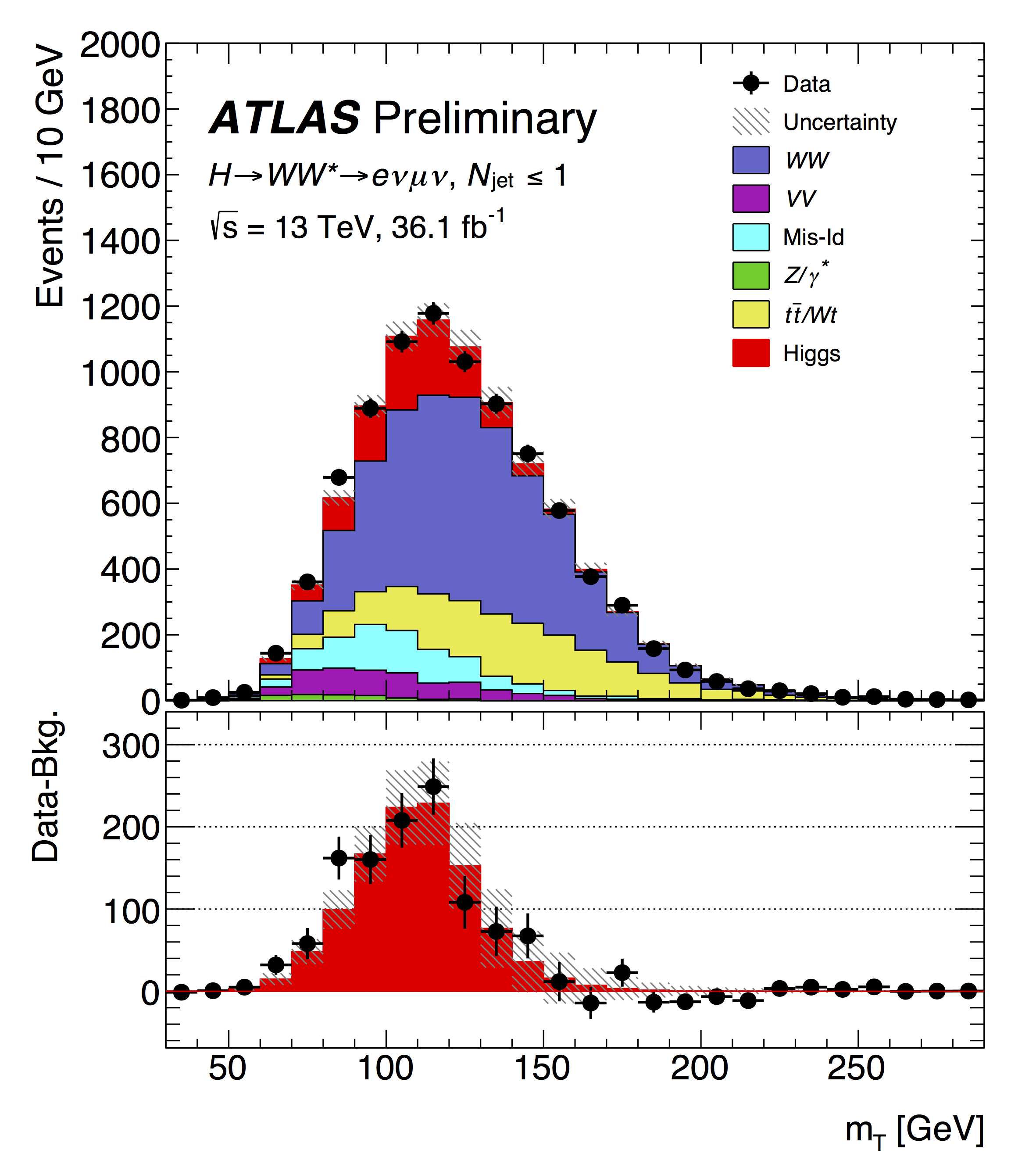Figure 1: The transverse mass of the selected Higgs-boson candidate events. The contribution from the Higgs boson is shown in red, the bottom panel compares the background-subtracted data points to the expected yield from the Higgs boson in the red histogram. (Image: ATLAS Collaboration/CERN)

Discovering the Higgs boson can be likened to finding a new continent. While a momentous event in itself, the most exciting part remains the exploration of the new land! Will physicists find what they expect, a Higgs boson which behaves in every way as predicted? Or will they uncover deviations from the predicted properties? Such deviations could indicate the existence of new particles or forces that interact with the Higgs boson, or even additional Higgs boson species.

In a new result presented today at the Rencontres de Moriond, the ATLAS collaboration examined the Higgs boson decaying into two W bosons (H→ W+W-), the charged force carriers of the weak interaction. The analysis uses 36 fb-1 of Run 2 data taken in 2015 and 2016 at a collision energy of 13 TeV. With a predicted decay probability (branching fraction) of about 21%, H→W+W- is the most common decay mode after the decay to b-quark pairs; yet it required a tour de force to achieve this result.

The present analysis is similar to earlier analyses carried out on the 7 and 8 TeV datasets from Run 1: it focuses on the most ubiquitous Higgs boson production modes, where either two gluons (ggF), or two W or Z bosons (VBF) emitted by the colliding protons fuse to produce the Higgs boson. The W bosons from the Higgs boson decay are unstable and immediately decay to lighter particles. Decays to electrons and muons are considered, which provide a cleaner signal than the more abundant decays to a pair of quarks, but which reduce the exploitable H→W+W- branching fraction to only 1.1%. An additional challenge is that these W boson decays are accompanied by invisible neutrinos, giving rise to missing energy in the event, thus making the signal reconstruction more challenging.

### With a predicted decay probability of about 21%, H→W+W- is the most common decay mode after the decay to b-quark pairs; yet it required a tour de force to achieve this result.Figure 2: The measured cross sections times branching fractions for Higgs boson decays to two W bosons for the gluon-gluon fusion (ggF) and vector boson fusion (VBF) production modes. The measured values are 12.3 +2.3 / -2.1 pb and 0.5 ± 0.3 pb, respectively, which are in agreement with precise theoretical calculations based on the Standard Model. The contours surrounding the measured values indicate the 68% and 95% confidence intervals, the standard model expectations (the red point in the plot) are compatible with the measured values. (Image: ATLAS Collaboration/CERN)

Data taking conditions are quite a bit harsher in Run 2, with increased beam intensity at the collision point leading to up to 60 simultaneous proton-proton interactions per bunch crossing. This made it much more difficult to distinguish the electrons and muons from W boson decays from other sources, and even from other particles. Refining the analysis proved a challenge – one that has now been met by ATLAS.

Figure 1 shows the so-called transverse mass of the events, computed from the visible and invisible decay products of the Higgs boson candidates. The red contribution is the expectation from the Higgs boson, and the other colours represent various background processes. The Higgs-boson signal, which amounts to a significance exceeding six standard deviations, is clearly visible in the figure. Figure 2 shows the measured cross sections times branching fractions for Higgs boson decays to two W bosons for the ggF and VBF production modes.

Such detailed studies of the Higgs boson with Run 2 data are an encouraging sign of things to come. The H→W+W- decay played an important role in the scrutiny of the Higgs boson’s nature with Run 1 data, and we expect it to carry its weight in the even more incisive tests that will be possible with the further Run 2 data.